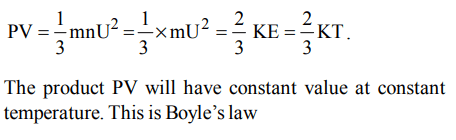## States of Matter Questions and Answers Part-3

1. Boyle’s law, according to kinetic equation can be expressed as
a) PV = KT
b) PV = RT
c) $PV=\frac{3}{2}kT$
d) $PV=\frac{2}{3}kT$

Explanation:2. The ratio between the root mean square velocity of $H_{2}$ at 50 K and that of $O_{2}$ at 800 K is
a) 4
b) 2
c) 1
d) 1/4

Explanation:3.The r.m.s velocity of hydrogen is $\sqrt{7}$ times the r.m.s velocity of nitrogen. If T is the temperature of the gas , than
a) $T\left(H_{2}\right)=T\left(N_{2}\right)$
b) $T\left(H_{2}\right)>T\left(N_{2}\right)$
c) $T\left(H_{2}\right)<T\left(N_{2}\right)$
d) $T\left(H_{2}\right)=\sqrt{7}\left(N_{2}\right)$

Explanation:4. The r.m.s velocity of $CO_{2}$ at temperature T (in kelvin) is $x cms^{-1}$ . At what temperature (in kelvin) the r.m.s. velocity of nitrous oxide would be $4x cms^{-1}$ ?
a) 16 T
b) 2 T
c) 4 T
d) 32 T

Explanation:5. Which of the following has maximum root mean square velocity at the same temperature?
a) $SO_{2}$
b) $CO_{2}$
c) $O_{2}$
d) $H_{2}$

Explanation: $U \propto \sqrt{\frac{1}{M}}$   hence hydrogen (molecular weight being the lowest) has the maximum root mean square velocity

6. Density ratio of $O_{2} and H_{2}$  is 16:1. The ratio of their r.m.s. velocities will be
a) 4 : 1
b) 1 : 16
c) 1 : 4
d) 16 : 1

Explanation: $\frac{U_{1}}{U_{2}}= \sqrt{\frac{d_{2}}{d_{1}}} = \sqrt{\frac{1}{16}} = 1:4$

7. At what temperature the RMS velocity of $SO_{2}$ be same as that of $O_{2}$ at 303 K ?
a) 273 K
b) 606 K
c) 303 K
d) 403 K

Explanation: Apply $\frac{U_{1}}{U_{2}} = \sqrt{\frac{T_{1}M_{2}}{T_{2}M_{1}}}$

8. The temperature of an ideal gas is reduced from 927ºC to 27ºC. the r.m.s. velocity of the molecules becomes
a) double the inital value
b) half of the initial value
c) four times the initial value
d) ten times the initial value

Explanation:9. If the average velocity of $N_{2}$ molecues is 0.3 m/s at 27ºC, then the velocity of 0.6 m/s will take place at
a) 273 K
b) 927 K
c) 1000 K
d) 1200 K